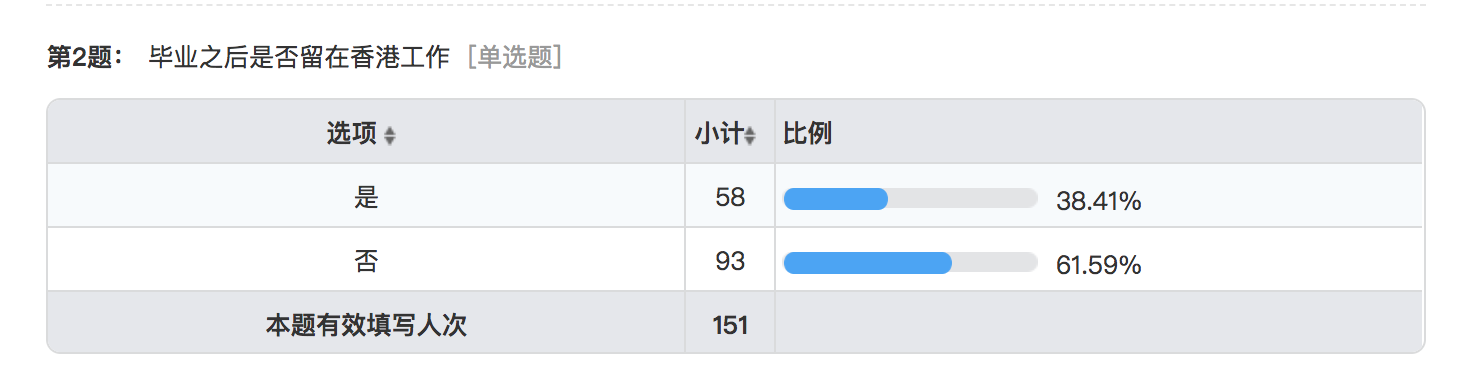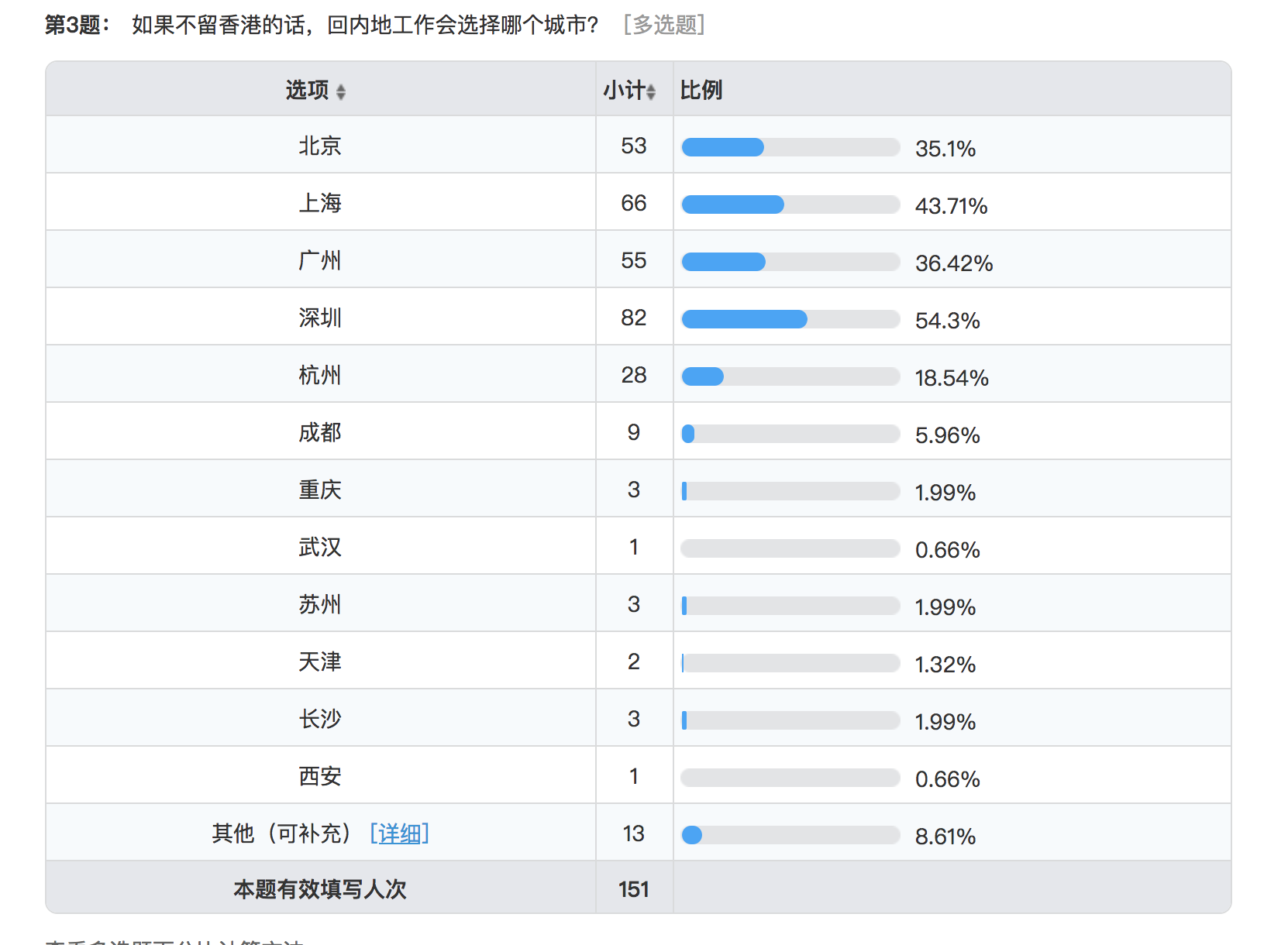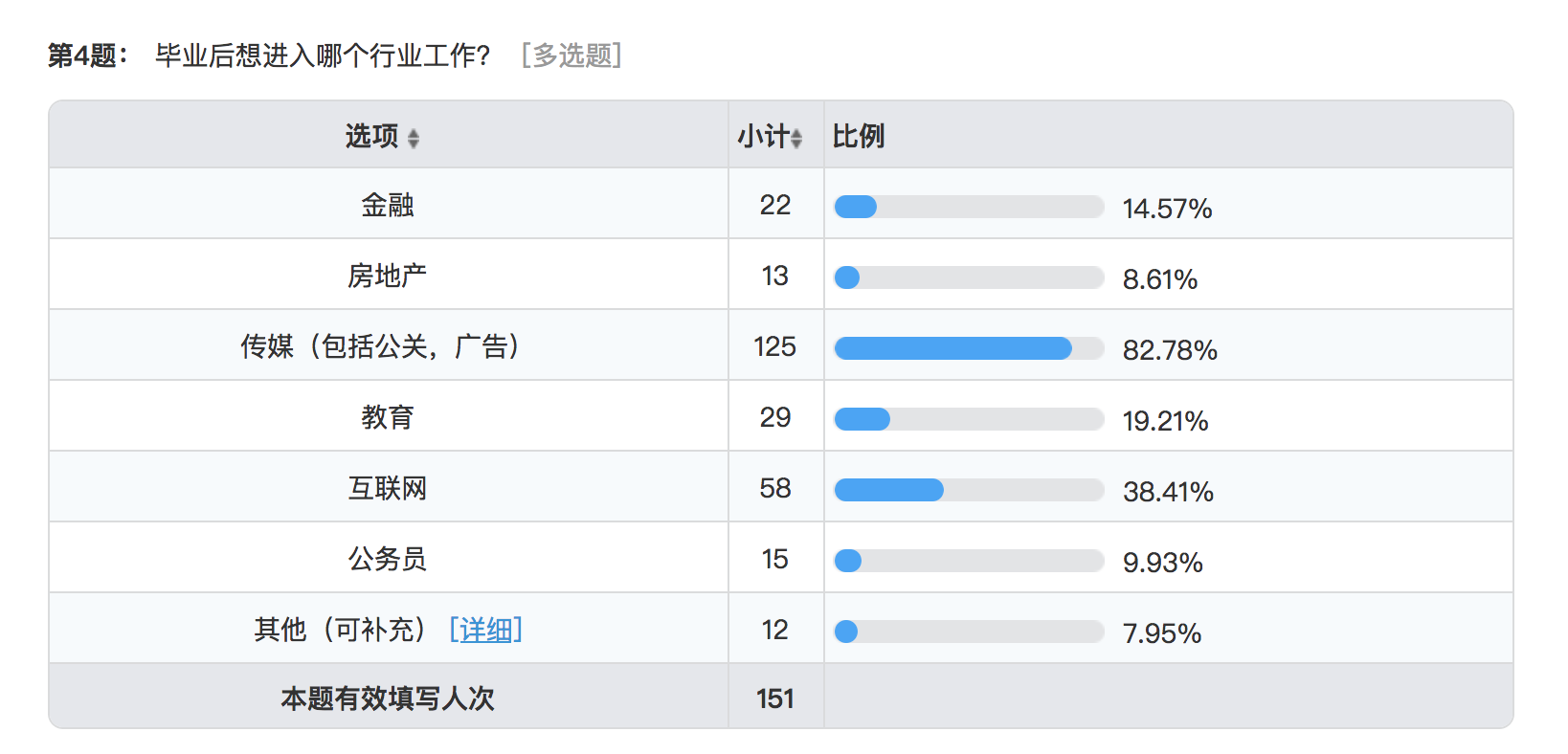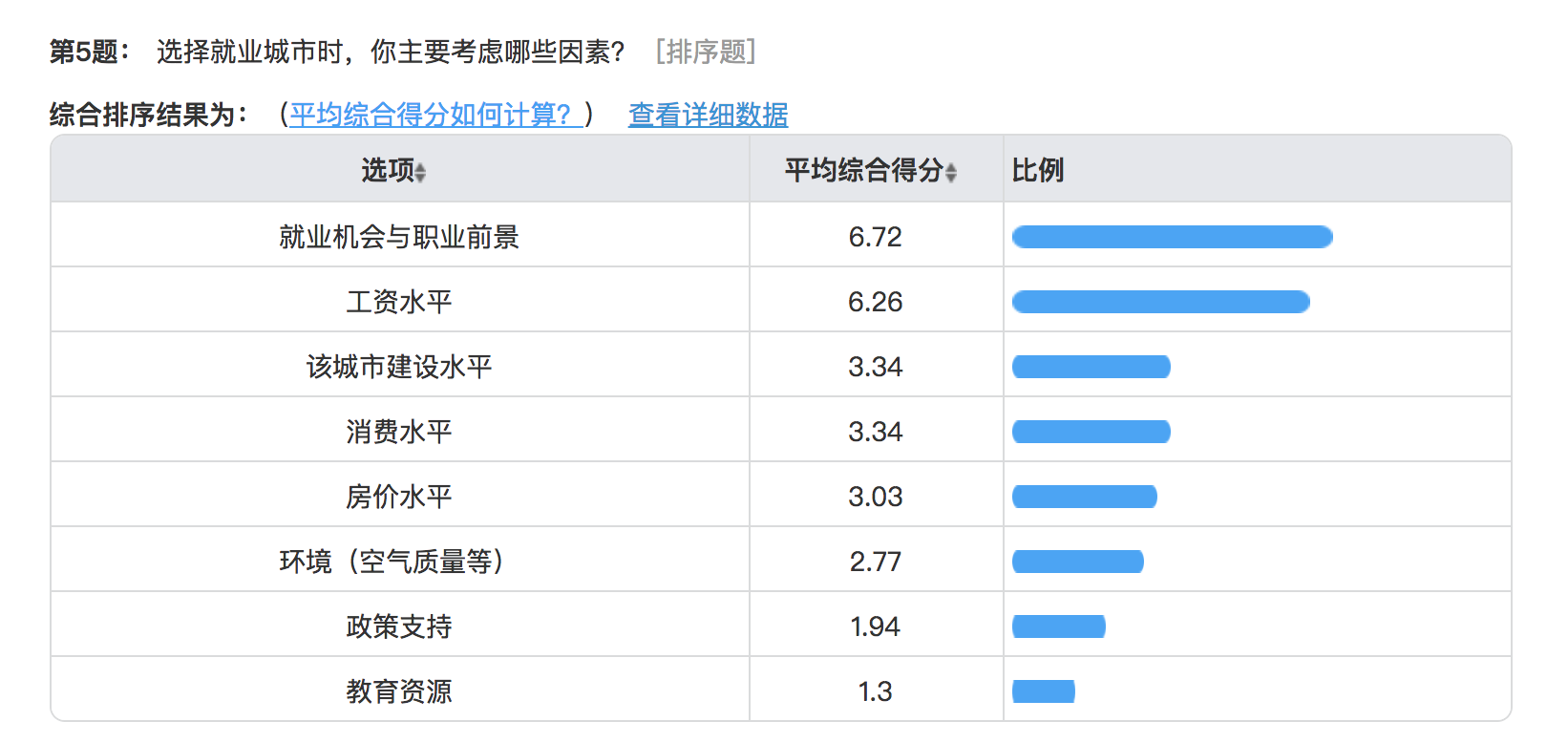In :
from IPython.core.display import Image
import pandas as pd
import matplotlib
import plotly
import seaborn as sns
from pandas import Series,DataFrame
import csv
import plotly.plotly as py
import plotly.graph_objs as go
plotly.offline.init_notebook_mode(connected=True)
from plotly.graph_objs import Scatter, Layout
import numpy as np
from plotly.graph_objs import Bar
from plotly.offline import iplot


Over the past five decades, School of Communication has nurtured more than 10,000 graduates, which could be a strong alumni network.However, our school has limited information on the career choices for graduates, since there is not an official survey to collect such statistics. In order to help our school to fulfill this task, we issued questionnaires across all post-graduate majors in School of Communication, targeting at graduate students who are about to obtain their master degree this fall. https://www.wjx.cn/m/22808330.aspx?from=groupmessage&isappinstalled=0 (Questionnaire)

In :
Image('留港與否.png')
# produced by 问卷星.cn, descriptive analysis
# graph name:留港與否.png,foler name: pics
# data file:浸会大学就业去向调查选项版.xls, folder name: excel_and_csv

Out:The result shows that more than 60 percent of students surveyed will leave Hong Kong and return back to mainland after graduation

In :
Image('問卷星_內地就業城市選擇.png')
# produced by 问卷星.cn, descriptive analysis
# graph name:.問卷星_內地就業城市選擇.png,foler name: pics
# data file:浸会大学就业去向调查选项版.xls, folder name: excel_and_csv

Out:China’s first-tier cities, Beijing, Shanghai, Guangzhou and Shenzhen, are still the most popular destinations for students. 54.3 percent of the students surveyed choose Shenzhen as the place where they would like to work most after graduation. Shanghai is the second favorite city of graduate students from School of Communication, with a share of 43.71 percent, followed by Guangzhou (36.42 percent). Beijing falls on the bottom of rankings among four first-tier cities, as 53 students surveyed choose it as the place to start their career, slightly 1.4 percent less than Shanghai. Hangzhou and Chengdu stands out among the remaining cities, each attracting 18.54 percent and 5.96 percent of graduate students.

In :
Image('問卷星_就業行業選擇.png')
# produced by 问卷星.cn, descriptive analysis
# graph name:.問卷星_就業行業選擇.png,foler name: pics
# data file:浸会大学就业去向调查选项版.xls, folder name: excel_and_csv

Out:Our survey also focused on graduate students’ career preference. It’s not surprising that more than four-fifths of students from School of Communication are willing to enter media industry after graduation, since all their majors and curriculum are closely related with it.

In :
Image('問卷星_就業城市考慮因素.png')
# produced by 问卷星.cn,
# The calculation methods of the average comprehensive score of the options(平均综合得分) is (sigma frequency * weight)/ fill-in number
# It reflects the comprehensive ranking of the options, and the higher the score is, the better the ranking is.
# graph name:.問卷星_就業城市考慮因素.png,foler name: pics
# data file:浸会大学就业去向调查选项版.xls, folder name: excel_and_csv

Out:When it comes to what affects their choices of city, there are two factors that students surveyed consider most: the employment opportunities and career prospects in a city; the industry’s average salary. These two factors decide directly that how much they can earn and whether they have more chance to get promoted after entering the workforce. In a word, all is about MONEY!

Since more than half of students surveyed plan to work in mainland China, particularly in big cities such as Beijing, Shanghai, Guangzhou, Shenzhen, Hangzhou and Chengdu, it would be great for them to have a better idea of the city they chose.

Part Two:The analysis of the top 6 cities

（1）Jobs & Salary

As we just mentioned in the first part, job prospect is the most important factor for newly graduates to choose their future destination. We want to further analyze the employment market for media industry in these six major cities in mainland China, so we choose the most popular job search website named 51job (https://search.51job.com/) as our data source. Media-related job skill, cities, full-time position, no previous experience are filter conditions when we were scraping the enormous information on the website. Then we found that there are over 15,000 data (around 2500 for each city) in total to support our analysis.

In :
import requests
from bs4 import BeautifulSoup
import csv

In :
url="https://search.51job.com/list/020000,000000,0000,12%252C48%252C49%252C13,9,99,%2520-%25E5%25AE%259E%25E4%25B9%25A0,2,1.html?lang=c&stype=&postchannel=0000&workyear=01&cotype=99&degreefrom=99&jobterm=99&companysize=99&providesalary=99&lonlat=0%2C0&radius=-1&ord_field=0&confirmdate=9&fromType=&dibiaoid=0&address=&line=&specialarea=00&from=&welfare="
r = requests.get(url)
mypage=BeautifulSoup(r.text)
r.encoding = 'gbk'

/Users/marla/Desktop/marla322/venv/lib/python3.6/site-packages/bs4/__init__.py:181: UserWarning: No parser was explicitly specified, so I'm using the best available HTML parser for this system ("lxml"). This usually isn't a problem, but if you run this code on another system, or in a different virtual environment, it may use a different parser and behave differently.

The code that caused this warning is on line 193 of the file /Library/Frameworks/Python.framework/Versions/3.6/lib/python3.6/runpy.py. To get rid of this warning, change code that looks like this:

BeautifulSoup(YOUR_MARKUP})

to this:

BeautifulSoup(YOUR_MARKUP, "lxml")

markup_type=markup_type))


we grab 3 types of information for every city: the position, the salary and the company. Here is the example of Shanghai, then we apply this part of code to ohter 5 cities.

In [ ]:
urls=[]
Total_position=[]
Total_company=[]
Total_salary=[]

for i in range (0,71):
urls.append(url)

for url in urls:
r =requests.get(url)
r.encoding = 'gbk'

mypage=BeautifulSoup(r.text,'lxml')

positions=mypage.find_all('p',attrs={'class':'t1'})
position=positions
for position in positions:
my_position=position.find('a').text.strip(' \t\n\r')
Total_position.append(my_position)

companies=mypage.find_all('span',attrs={'class':'t2'})
company=companies
for company in companies:
Total_company.append(company.text)

salaries=mypage.find_all('span',attrs={'class':'t4'})
salary=salaries
for salary in salaries:
Total_salary.append(salary.text)

Total_company=Total_company[1:]
Total_salary=Total_salary[1:]

In [ ]:
# （since the units of measure of salary are different, we did some adjustment on that)
final_salary=[]
Total_salary
for the_salary in Total_salary:
all_salary=the_salary#.split()
#print(all_salary)

if all_salary[-3:] == '千/月':
salary=(all_salary[:-3])
salary = float(salary.split('-')) * 1000
final_salary.append(salary)
#break
elif all_salary[-3:] == '万/月':
salary=(all_salary[:-3])
salary = float(salary.split('-')) * 10000
final_salary.append(salary)
elif all_salary[-3:] == '万/年':
salary=(all_salary[:-3])
salary = float(salary.split('-'))/12*10000
final_salary.append(salary)
elif all_salary[-3:] == '元/天':
salary=(all_salary[:-3])
salary = float(salary.split('-'))*22
final_salary.append(salary)

else:
salary = 0
final_salary.append(salary)

In :
with open("Shanghai.csv",'w',newline='') as f:
spamwriter = csv.writer(f)
spamwriter.writerows(Total_position)


Generally, Media-related job skill here comprises: • content generated direction, including reporter, editor and advertisement participants; • marketing & communication direction, including PR, marketing, promoting, market research and sales participants; • other related

In :
# Take Shenzhen as example.we use these key words to search these jobs and count the total occupation number in each category and the average salary. Then apply this logic to other 5 cities
df_content_generator=df['Total_position'].apply(lambda t:'记者' in str(t))|df['Total_position'].apply(lambda t:'文案' in str(t))|df['Total_position'].apply(lambda t:'编辑' in str(t))|df['Total_position'].apply(lambda t:'广告' in str(t))|df['Total_position'].apply(lambda t:'文化传播' in str(t))
content_position=df_content_generator.aggregate('sum')
contend_salary_mean=df[df_content_generator].mean()
df_marketing=df['Total_position'].apply(lambda t:'公关' in str(t))|df['Total_position'].apply(lambda t:'推广' in str(t))|df['Total_position'].apply(lambda t:'市场研究' in str(t))|df['Total_position'].apply(lambda t:'销售' in str(t))|df['Total_position'].apply(lambda t:'策划' in str(t))
marketing_position=df_marketing.aggregate('sum')
marketing_salary_mean=df[df_marketing].mean()
df_other=df['Total_position'].apply(lambda t:'行政' in str(t))|df['Total_position'].apply(lambda t:'文员' in str(t))
other_position=df_other.aggregate('sum')
other_salary_mean=df[df_other].mean()

df_content_generator=df['Total_position'].apply(lambda t:'记者' in str(t))|df['Total_position'].apply(lambda t:'文案' in str(t))|df['Total_position'].apply(lambda t:'编辑' in str(t))|df['Total_position'].apply(lambda t:'广告' in str(t))|df['Total_position'].apply(lambda t:'文化传播' in str(t))
shanghai_content_position=df_content_generator.aggregate('sum')
shanghai_contend_salary_mean=df[df_content_generator].mean()
df_marketing=df['Total_position'].apply(lambda t:'公关' in str(t))|df['Total_position'].apply(lambda t:'推广' in str(t))|df['Total_position'].apply(lambda t:'市场研究' in str(t))|df['Total_position'].apply(lambda t:'销售' in str(t))|df['Total_position'].apply(lambda t:'策划' in str(t))
shanghai_marketing_position=df_marketing.aggregate('sum')
shanghai_marketing_salary_mean=df[df_marketing].mean()
df_other=df['Total_position'].apply(lambda t:'行政' in str(t))|df['Total_position'].apply(lambda t:'文员' in str(t))
shanghai_other_position=df_other.aggregate('sum')
shanghai_other_salary_mean=df[df_other].mean()

df_content_generator=df['Total_position'].apply(lambda t:'记者' in str(t))|df['Total_position'].apply(lambda t:'文案' in str(t))|df['Total_position'].apply(lambda t:'编辑' in str(t))|df['Total_position'].apply(lambda t:'广告' in str(t))|df['Total_position'].apply(lambda t:'文化传播' in str(t))
guangzhou_content_position=df_content_generator.aggregate('sum')
guangzhou_contend_salary_mean=df[df_content_generator].mean()
df_marketing=df['Total_position'].apply(lambda t:'公关' in str(t))|df['Total_position'].apply(lambda t:'推广' in str(t))|df['Total_position'].apply(lambda t:'市场研究' in str(t))|df['Total_position'].apply(lambda t:'销售' in str(t))|df['Total_position'].apply(lambda t:'策划' in str(t))
guangzhou_marketing_position=df_marketing.aggregate('sum')
guangzhou_marketing_salary_mean=df[df_marketing].mean()
df_other=df['Total_position'].apply(lambda t:'行政' in str(t))|df['Total_position'].apply(lambda t:'文员' in str(t))
guangzhou_other_position=df_other.aggregate('sum')
guangzhou_other_salary_mean=df[df_other].mean()

df_content_generator=df['Total_position'].apply(lambda t:'记者' in str(t))|df['Total_position'].apply(lambda t:'文案' in str(t))|df['Total_position'].apply(lambda t:'编辑' in str(t))|df['Total_position'].apply(lambda t:'广告' in str(t))|df['Total_position'].apply(lambda t:'文化传播' in str(t))
Hangzhou_content_position=df_content_generator.aggregate('sum')
Hangzhou_contend_salary_mean=df[df_content_generator].mean()
df_marketing=df['Total_position'].apply(lambda t:'公关' in str(t))|df['Total_position'].apply(lambda t:'推广' in str(t))|df['Total_position'].apply(lambda t:'市场研究' in str(t))|df['Total_position'].apply(lambda t:'销售' in str(t))|df['Total_position'].apply(lambda t:'策划' in str(t))
Hangzhou_marketing_position=df_marketing.aggregate('sum')
Hangzhou_marketing_salary_mean=df[df_marketing].mean()
df_other=df['Total_position'].apply(lambda t:'行政' in str(t))|df['Total_position'].apply(lambda t:'文员' in str(t))
Hangzhou_other_position=df_other.aggregate('sum')
Hangzhou_other_salary_mean=df[df_other].mean()

df_content_generator=df['Total_position'].apply(lambda t:'记者' in str(t))|df['Total_position'].apply(lambda t:'文案' in str(t))|df['Total_position'].apply(lambda t:'编辑' in str(t))|df['Total_position'].apply(lambda t:'广告' in str(t))|df['Total_position'].apply(lambda t:'文化传播' in str(t))
chengdu_content_position=df_content_generator.aggregate('sum')
Chengdu_contend_salary_mean=df[df_content_generator].mean()
df_marketing=df['Total_position'].apply(lambda t:'公关' in str(t))|df['Total_position'].apply(lambda t:'推广' in str(t))|df['Total_position'].apply(lambda t:'市场研究' in str(t))|df['Total_position'].apply(lambda t:'销售' in str(t))|df['Total_position'].apply(lambda t:'策划' in str(t))
Chengdu_marketing_position=df_marketing.aggregate('sum')
Chengdu_marketing_salary_mean=df[df_marketing].mean()
df_other=df['Total_position'].apply(lambda t:'行政' in str(t))|df['Total_position'].apply(lambda t:'文员' in str(t))
Chengdu_other_position=df_other.aggregate('sum')
Chengdu_other_salary_mean=df[df_other].mean()

df_content_generator=df['Total_position'].apply(lambda t:'记者' in str(t))|df['Total_position'].apply(lambda t:'文案' in str(t))|df['Total_position'].apply(lambda t:'编辑' in str(t))|df['Total_position'].apply(lambda t:'广告' in str(t))|df['Total_position'].apply(lambda t:'文化传播' in str(t))
Beijing_content_position=df_content_generator.aggregate('sum')
Beijing_contend_salary_mean=df[df_content_generator].mean()
df_marketing=df['Total_position'].apply(lambda t:'公关' in str(t))|df['Total_position'].apply(lambda t:'推广' in str(t))|df['Total_position'].apply(lambda t:'市场研究' in str(t))|df['Total_position'].apply(lambda t:'销售' in str(t))|df['Total_position'].apply(lambda t:'策划' in str(t))
Beijing_marketing_position=df_marketing.aggregate('sum')
Beijing_marketing_salary_mean=df[df_marketing].mean()
df_other=df['Total_position'].apply(lambda t:'行政' in str(t))|df['Total_position'].apply(lambda t:'文员' in str(t))
Beijing_other_position=df_other.aggregate('sum')
Beijing_other_salary_mean=df[df_other].mean()

In :
trace1 = go.Bar(
y=['other related', 'marketing', 'content<br>generation'],
x=[Beijing_other_position, Beijing_marketing_position, Beijing_content_position],
name='Beijing',
orientation = 'h',
marker = dict(
color = 'rgb(255, 255, 204)',
line = dict(
color = 'rgb(255, 255, 204)',
width = 3)
)
)
trace2 = go.Bar(
y=['other related', 'marketing', 'content<br>generation'],
x=[shanghai_other_position, shanghai_marketing_position, shanghai_content_position],
name='Shanghai',
orientation = 'h',
marker = dict(
color = 'rgb(161, 218, 180)',
line = dict(
color = 'rgb(161, 218, 180)',
width = 3)
)
)
trace3 = go.Bar(
y=['other related', 'marketing', 'content<br>generation'],
x=[guangzhou_other_position, guangzhou_marketing_position, guangzhou_content_position],
name='Guangzhou',
orientation = 'h',
marker = dict(
color = 'rgb(65, 182, 196)',
line = dict(
color = 'rgb(65, 182, 196)',
width = 3)
)
)
trace4 = go.Bar(
y=['other related', 'marketing', 'content<br>generation'],
x=[other_position, marketing_position, content_position],
name='Shenzhen',
orientation = 'h',
marker = dict(
color = 'rgb(44, 127, 184)',
line = dict(
color = 'rgb(44, 127, 184)',
width = 3)
)
)
trace5 = go.Bar(
y=['other related', 'marketing', 'content<br>generation'],
x=[Hangzhou_other_position, Hangzhou_marketing_position, Hangzhou_content_position],
name='Hangzhou',
orientation = 'h',
marker = dict(
color = 'rgb(8, 104, 172)',
line = dict(
color = 'rgb(8, 104, 172)',
width = 3)
)
)
trace6 = go.Bar(
y=['other related', 'marketing', 'content<br>generation'],
x=[Chengdu_other_position, Chengdu_marketing_position, chengdu_content_position],
name='Chengdu',
orientation = 'h',
marker = dict(
color = 'rgb(37, 52, 148)',
line = dict(
color = 'rgb(37, 52, 148)',
width = 3)
)
)

data = [trace1, trace2,trace3,trace4,trace5,trace6]
layout = {
"barmode": "stack",
"title": "Communication&Media Related Jobs",
"xaxis": {
"autorange": True,
"range": [0, 4573.68421053],
"title": "Click to enter X axis title",
"type": "linear"
},
"yaxis": {
"autorange": True,
"range": [-0.5, 2.5],
"type": "category"
}
}

fig = go.Figure(data=data, layout=layout)
iplot(fig, filename='marker-h-bar')


From the chart above, in terms of job skill, we can simply find that the amount of the marketing-related job dominate the job market, which surpasses the sum of content-generated jobs and other jobs. And at the same time, the marketing job vacancies in Guangzhou staying at 1325 units occupy the most part, followed by Shanghai (745 units )and Shenzhen (633 units). As for total job amount in different cities, the situation among the first tier cities including Beijing, Shanghai, Guangzhou and Shenzhen are more competitive and attractive than that of Hangzhou and Chengdu.

In order to have a better idea of what kind of media-related job is mostly needed in six cities, we subdivide these three categories(content-generated, marketing, other related) into eleven sub-categories based on keywords by scraping information from the data we have:

• Content-generated: journalist, editor, copywriter, designer • Marketing: advertiser, PR, promotor, salesperson • Other-related: consultant

In :
df=pd.read_csv('Guangzhou_51job.csv', encoding= 'gb18030')
df_df=df['Total_position'].apply(lambda t:'实习' not in str(t))
df_g=df[df_df]
df_g_repo=df_g['Total_position'].apply(lambda t:'记者' in str(t)).aggregate('sum')
df_g_edi=df_g['Total_position'].apply(lambda t:'编辑' in str(t)).aggregate('sum')
df_g_ch=df_g['Total_position'].apply(lambda t:'策划' in str(t)).aggregate('sum')
df_g_sj=df_g['Total_position'].apply(lambda t:'设计' in str(t)).aggregate('sum')
df_g_pr=df_g['Total_position'].apply(lambda t:'公关' in str(t)).aggregate('sum')
df_g_yx=df_g['Total_position'].apply(lambda t:'营销' in str(t)).aggregate('sum')
df_g_tg=df_g['Total_position'].apply(lambda t:'推广' in str(t)).aggregate('sum')
df_g_zx=df_g['Total_position'].apply(lambda t:'咨询' in str(t)).aggregate('sum')

df_df=df['Total_position'].apply(lambda t:'实习' not in str(t))
df_s=df[df_df]
df_s_repo=df_s['Total_position'].apply(lambda t:'记者' in str(t)).aggregate('sum')
df_s_edi=df_s['Total_position'].apply(lambda t:'编辑' in str(t)).aggregate('sum')
df_s_ch=df_s['Total_position'].apply(lambda t:'策划' in str(t)).aggregate('sum')
df_s_sj=df_s['Total_position'].apply(lambda t:'设计' in str(t)).aggregate('sum')
df_s_pr=df_s['Total_position'].apply(lambda t:'公关' in str(t)).aggregate('sum')
df_s_yx=df_s['Total_position'].apply(lambda t:'营销' in str(t)).aggregate('sum')
df_s_tg=df_s['Total_position'].apply(lambda t:'推广' in str(t)).aggregate('sum')
df_s_zx=df_s['Total_position'].apply(lambda t:'咨询' in str(t)).aggregate('sum')

df_df=df['Total_position'].apply(lambda t:'实习' not in str(t))
df_H=df[df_df]
df_H_reporter=df_H['Total_position'].apply(lambda t:'记者' in str(t)).aggregate('sum')
df_H_edi=df_H['Total_position'].apply(lambda t:'编辑' in str(t)).aggregate('sum')
df_H_pn=df_H['Total_position'].apply(lambda t:'策划' in str(t)).aggregate('sum')
df_H_ds=df_H['Total_position'].apply(lambda t:'设计' in str(t)).aggregate('sum')
df_H_pr=df_H['Total_position'].apply(lambda t:'公关' in str(t)).aggregate('sum')
df_H_mk=df_H['Total_position'].apply(lambda t:'推广' in str(t)).aggregate('sum')
df_H_yx=df_H['Total_position'].apply(lambda t:'营销' in str(t)).aggregate('sum')
df_H_zx=df_H['Total_position'].apply(lambda t:'咨询' in str(t)).aggregate('sum')

df_df=df['Total_position'].apply(lambda t:'实习' not in str(t))
df_C=df[df_df]
df_C_reporter=df_C['Total_position'].apply(lambda t:'记者' in str(t)).aggregate('sum')
df_C_edi=df_C['Total_position'].apply(lambda t:'编辑' in str(t)).aggregate('sum')
df_C_pn=df_C['Total_position'].apply(lambda t:'策划' in str(t)).aggregate('sum')
df_C_ds=df_C['Total_position'].apply(lambda t:'设计' in str(t)).aggregate('sum')
df_C_pr=df_C['Total_position'].apply(lambda t:'公关' in str(t)).aggregate('sum')
df_C_mk=df_C['Total_position'].apply(lambda t:'推广' in str(t)).aggregate('sum')
df_C_yx=df_C['Total_position'].apply(lambda t:'营销' in str(t)).aggregate('sum')
df_C_zx=df_C['Total_position'].apply(lambda t:'咨询' in str(t)).aggregate('sum')

df_df=df['Total_position'].apply(lambda t:'实习' not in str(t))
df_B=df[df_df]
df_B_reporter=df_B['Total_position'].apply(lambda t:'记者' in str(t)).aggregate('sum')
df_B_edi=df_B['Total_position'].apply(lambda t:'编辑' in str(t)).aggregate('sum')
df_B_pn=df_B['Total_position'].apply(lambda t:'策划' in str(t)).aggregate('sum')
df_B_ds=df_B['Total_position'].apply(lambda t:'设计' in str(t)).aggregate('sum')
df_B_pr=df_B['Total_position'].apply(lambda t:'公关' in str(t)).aggregate('sum')
df_B_mk=df_B['Total_position'].apply(lambda t:'推广' in str(t)).aggregate('sum')
df_B_yx=df_B['Total_position'].apply(lambda t:'营销' in str(t)).aggregate('sum')
df_B_zx=df_B['Total_position'].apply(lambda t:'咨询' in str(t)).aggregate('sum')

df_df=df['Total_position'].apply(lambda t:'实习' not in str(t))
df_SH=df[df_df]
df_SH_reporter=df_SH['Total_position'].apply(lambda t:'记者' in str(t)).aggregate('sum')
df_SH_edi=df_SH['Total_position'].apply(lambda t:'编辑' in str(t)).aggregate('sum')
df_SH_pn=df_SH['Total_position'].apply(lambda t:'策划' in str(t)).aggregate('sum')
df_SH_ds=df_SH['Total_position'].apply(lambda t:'设计' in str(t)).aggregate('sum')
df_SH_pr=df_SH['Total_position'].apply(lambda t:'公关' in str(t)).aggregate('sum')
df_SH_mk=df_SH['Total_position'].apply(lambda t:'推广' in str(t)).aggregate('sum')
df_SH_yx=df_SH['Total_position'].apply(lambda t:'营销' in str(t)).aggregate('sum')
df_SH_zx=df_SH['Total_position'].apply(lambda t:'咨询' in str(t)).aggregate('sum')

In :
trace = go.Heatmap(z=[[df_B_reporter, df_B_edi, df_B_pn, df_B_ds, df_B_ad,df_B_pr,df_B_mk,df_B_yx,df_B_zx],
y=['Beijing', 'Shanghai','Guangzhou','Shenzhen','Hangzhou','Chengdu'])

data=[trace]
iplot(data, filename='labelled-heatmap')


From the heatmap above, it’s clear that jobs of design are all aggregated in four first-tier cities, particularly Guangzhou, Shenzhen and Shanghai. Beijing has a stronger need for occupations of editor and copywriter compared with other cities.

In terms of marketing-related work, students who plan to work as advertiser might have better career opportunities in Guangzhou, since the number of advertisement-related jobs there is far more than others. Promotion and sales are more favored in Beijing and Guangzhou.

One interesting point is, the needs for media-related jobs in Shenzhen, the city which is most popular for graduate students in School of Communication, actually are weaker than other three first-tier cities. Beijing and Guangzhou are two of the most friendly cities for communication graduates, according to our research

Salary level is another significant part of a city’s attraction for overseas graduates.So another part of our project is the average salary comparison among six top tier cities.

In :
trace1 = {
"x": ["content generation", "marketing", "other related"],
"y": [float(Beijing_contend_salary_mean),float(Beijing_marketing_salary_mean),float(Beijing_other_salary_mean)],
"marker": {"color": "rgb(255, 255, 204)"},
"name": "Beijing",
"type": "bar",
"uid": "fe7f98",
"xsrc": "XinyuM:3:cee1e4",
"ysrc": "XinyuM:3:58dd98"
}
trace2 = {
"x": ["content generation", "marketing", "other related"],
"y": [float(shanghai_contend_salary_mean),float(shanghai_marketing_salary_mean),float(shanghai_other_salary_mean)],
"marker": {"color": "rgb(161, 218, 180)"},
"name": "shanghai",
"type": "bar",
"uid": "a03931",
"xsrc": "XinyuM:3:cee1e4",
"ysrc": "XinyuM:3:23f69a"
}
trace3 = {
"x": ["content generation", "marketing", "other related"],
"y": [float(guangzhou_contend_salary_mean),float(guangzhou_marketing_salary_mean),float(guangzhou_other_salary_mean)],
"marker": {"color": "rgb(65, 182, 196)"},
"name": "Guangzhou",
"type": "bar",
"uid": "2ff10b",
"xsrc": "XinyuM:3:cee1e4",
}
trace4 = {
"x": ["content generation", "marketing", "other related"],
"y": [float(contend_salary_mean), float(marketing_salary_mean),float(other_salary_mean)],
"marker": {"color": "rgb(44, 127, 184)"},
"name": "shenzhen",
"type": "bar",
"uid": "f25fae",
"xsrc": "XinyuM:3:cee1e4",
"ysrc": "XinyuM:3:ee8231"
}
trace5 = {
"x": ["content generation", "marketing", "other related"],
"y": [float(Hangzhou_contend_salary_mean),float(Hangzhou_marketing_salary_mean),float(Hangzhou_other_salary_mean)],
"marker": {"color": "rgb(8, 104, 172)"},
"name": "Hangzhou",
"type": "bar",
"uid": "e55ccc",
"xsrc": "XinyuM:3:cee1e4",
"ysrc": "XinyuM:3:6a7458"
}
trace6 = {
"x": ["content generation", "marketing", "other related"],
"y": [float(Chengdu_contend_salary_mean),float(Chengdu_marketing_salary_mean),float(Chengdu_other_salary_mean)],
"marker": {"color": "rgb(37, 52, 148)"},
"name": "Chengdu",
"type": "bar",
"uid": "27f905",
"xsrc": "XinyuM:3:cee1e4",
"ysrc": "XinyuM:3:3b7d85"
}
data =[trace1, trace2, trace3, trace4, trace5, trace6]
layout = {
"annotations": [
{
"x": 0.0762331838565,
"y": 5439.80314391,
"arrowsize": 1,
"ax": 0,
"ay": -32,
"font": {
"color": "rgb(128, 0, 128)",
"size": 15
},
"showarrow": True,
"text": "<b>Shenzhen:5388.49RMB</b>",
"xref": "x",
"yref": "y"
},
{
"x": 0.80269058296,
"y": 5876.40339625,
"ax": 0,
"ay": -52,
"font": {
"color": "rgb(128, 0, 128)",
"size": 15
},
"showarrow": True,
"text": "<b>Shanghai:5832.25RMB</b>",
"xref": "x",
"yref": "y"
},
{
"x": 2.07623318386,
"y": 5677.79731725,
"ax": 0,
"ay": -42,
"font": {
"color": "rgb(128, 0, 128)",
"size": 15
},
"showarrow": True,
"text": "<b>Shenzhen:5647.52RMB</b>",
"xref": "x",
"yref": "y"
}
],
"barmode": "group",
"title": "Average Salary in 6 Cities",
"xaxis": {
"autorange": True,
"range": [-0.5, 2.5],
"tickangle": -25,
"title": "Position",
"type": "category"
},
"yaxis": {
"autorange": True,
"range": [0, 7383.47305453],
"title": "Salary",
"type": "linear"
}
}
fig = go.Figure(data=data, layout=layout)
iplot(fig, filename='angled-text-bar')


As we can see in the histogram above, Shenzhen ranks the first in the salary level for content-generated jobs and other jobs among six cities, reaching at 5,388.49 yuan and 5,647.52 yuan. And at the same time, Shanghai offers the most competitive wage for entry-level marketing jobs at 5,832.25 yuan, following by Hangzhou and Shenzhen. Interestingly, it seems Hangzhou performs very attractive in all three job categories.

(2)Housing Price:

Imagine when you step into a new city, which is far from your family and friends, how can you gain spiritual fulfillment? The answer is finding your own house, no matter is buying or renting. Based on this idea, we try to discuss the housing price in top tier cities and our source is a real estate agent website called An Juke (https://hangzhou.anjuke.com/) covering most part of China.

In :
df = pd.read_excel('6城市近5年商品房销售平均价格.xlsx')

In :
trace1 = go.Scatter(
x=df.index,
y=df['北京'].values,
name = 'Beijing',
marker=dict(
color='rgb(255, 255, 204)',
)
)
trace2 = go.Scatter(
x=df.index,
y=df['上海'].values,
name = 'Shanghai',
marker=dict(
color='rgb(161, 218, 180)',
)
)
trace3 = go.Scatter(
x=df.index,
y=df['广州'].values,
name = 'Guangzhou',
marker=dict(
color='rgb(65, 182, 196)',
)
)
trace4 = go.Scatter(
x=df.index,
y=df['深圳'].values,
name = 'Shenzhen',
marker=dict(
color='rgb(44, 127, 184)',
)
)
trace5 = go.Scatter(
x=df.index,
y=df['杭州'].values,
name = 'Hangzhou',
marker=dict(
color='rgb(8, 104, 172)',
)
)
trace6 = go.Scatter(
x=df.index,
y=df['成都'].values,
name = 'Chengdu',
marker=dict(
color='rgb(37, 52, 148)',
)
)

data = [trace1, trace2,trace3,trace4,trace5,trace6]
iplot({"data":data,
"layout": Layout(title="Average Commercial Housing Price from 2012-2016")
})


Among these cities, Beijing, Shanghai and Shenzhen still stand for the top three places in mainland China from 2012 to 2017 with some internal ranking changes. Guangzhou, Hangzhou and Chengdu experience higher speed increase during this period. Hangzhou’s average housing price climbed over 20,000 yuan due to the G20 summit and the momentum of technological companies becoming the new first and half tier city.

However, how to quantify the comparison between housing price and income level is very hard in reality. One effective way to measure this is housing price-to-income ratio. Here, we introduce a formula which fit in our scenario most.

Housing Price-to-Income Ratio=(80 square meterAverage Housing Price)/(Average Monthly Wage12)

We use 80 square meters to represent the house place because this size is suit for single youngsters generally.

In :
Beijing_per_year=(Beijing_contend_salary_mean+Beijing_marketing_salary_mean+Beijing_other_salary_mean)/3*12
Beijing_rate=54559*80/Beijing_per_year
Shanghai_per_year=(shanghai_contend_salary_mean+shanghai_marketing_salary_mean+shanghai_contend_salary_mean)/3*12
Shanghai_rate=50527*80/Shanghai_per_year
Guangzhou_per_year=(guangzhou_contend_salary_mean+guangzhou_marketing_salary_mean+guangzhou_other_salary_mean)/3*12
Guangzhou_rate=28586*80/Guangzhou_per_year
Shenzhen_per_year=(contend_salary_mean+marketing_salary_mean+other_salary_mean)/3*12
Shenzhen_rate=48997*80/Shenzhen_per_year
Hangzhou_per_year=(Hangzhou_contend_salary_mean+Hangzhou_marketing_salary_mean+Hangzhou_other_salary_mean)/3*12
Hangzhou_rate=20930*80/Hangzhou_per_year
Chengdu_per_year=(Chengdu_contend_salary_mean+Chengdu_marketing_salary_mean+Chengdu_other_salary_mean)/3*12
Chengdu_rate=13023*80/Chengdu_per_year

In :
from plotly import tools

import numpy as np

y_saving = [float(Chengdu_rate),float(Hangzhou_rate),float(Guangzhou_rate),float(Shenzhen_rate),
float(Shanghai_rate), float(Beijing_rate)]
y_net_worth = [13023, 20930,28586,48997,50527, 54559]
x_saving = ['Chengdu', 'Hangzhou', 'Guangzhou', 'Shengzhen',
'Shanghai', 'Beijing']
x_net_worth = ['Chengdu', 'Hangzhou','Guangzhou','Shengzhen',
'Shanghai', 'Beijing']
trace0 = go.Bar(
x=y_saving,
y=x_saving,
marker=dict(
color='rgb(37, 52, 148)',
line=dict(
color='rgb(37, 52, 148)',
width=1),
),
name='HousingPrice-to-Income Ratio',
orientation='h',
)
trace1 = go.Scatter(
x=y_net_worth,
y=x_net_worth,
mode='lines+markers',
line=dict(
color='rgb(128, 0, 128)'),
name='Average commercial housing price on Apri 2018',
)
layout = dict(
title='HousingPrice-to-Income Ratio & Average Housing Price',
yaxis1=dict(
showgrid=False,
showline=False,
showticklabels=True,
domain=[0, 0.85],
),
yaxis2=dict(
showgrid=False,
showline=True,
showticklabels=False,
linecolor='rgba(102, 102, 102, 0.8)',
linewidth=2,
domain=[0, 0.85],
),
xaxis1=dict(
zeroline=False,
showline=False,
showticklabels=True,
showgrid=True,
domain=[0, 0.42],
),
xaxis2=dict(
zeroline=False,
showline=False,
showticklabels=True,
showgrid=True,
domain=[0.47, 1],
side='top',
dtick=25000,
),
legend=dict(
x=0.029,
y=1.038,
font=dict(
size=10,
),
),
margin=dict(
l=100,
r=20,
t=70,
b=70,
),
paper_bgcolor='rgb(253, 253, 253)',
plot_bgcolor='rgba(252, 252, 252, 0.06)',
)

annotations = []

y_s = np.round(y_saving, decimals=2)
y_nw = np.rint(y_net_worth)

for ydn, yd, xd in zip(y_nw, y_s, x_saving):

annotations.append(dict(xref='x2', yref='y2',
y=xd, x=ydn - 20000,
text='{:,}'.format(ydn) + 'RMB',
font=dict(family='Arial', size=12,
color='rgb(128, 0, 128)'),
showarrow=False))

annotations.append(dict(xref='x1', yref='y1',
y=xd, x=yd + 5,
text=str(yd),
font=dict(family='Arial', size=12,
color='rgb(37, 52, 148)'),
showarrow=False))

layout['annotations'] = annotations

fig = tools.make_subplots(rows=1, cols=2, specs=[[{}, {}]], shared_xaxes=True,
shared_yaxes=False, vertical_spacing=0.001)

fig.append_trace(trace0, 1, 1)
fig.append_trace(trace1, 1, 2)

fig['layout'].update(layout)
iplot(fig, filename='oecd-networth-saving-bar-line')

This is the format of your plot grid:
[ (1,1) x1,y1 ]  [ (1,2) x2,y2 ]



We could simply make a city ranking list in terms of the difficulty of buying a house from city to city. The higher the ratio score, the more difficult it is for people to purchase a property in a city. From the bar chart above, we can see the housing price-to-income ratio scores among these six cities, which indicates that Beijing (69.22) is the city where people are least likely to buy a house given current average wage level, followed by Shanghai (61.87) and Shenzhen(59.97). Chengdu is the most affordable city in terms of ratio scores.

(3)Talent Attaction Policy

In :
Image('Talent Attraction Policies.png')
# Firstly we graded 6 cities' talent attaction policy by evaluating their performance on 6 aspects you see on the graph based on offical documents we search.
# Then we made a excel file "人才引进政策数据.XLSX" that contains our grades and detialed policies as well
# use bdp.cn, a website that can do data visulization, import the file 人才引进政策数据.XLSX and do some adjustment online
# graph name: Talent Attraction Policies, folder name: pics
# date file:人才引进政策数据.XLSX， folder name: excel_and_csv

Out: